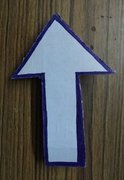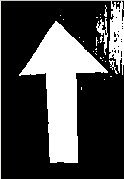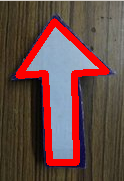# Python | Detect Polygons in an Image using OpenCV

Approach : The approach we would be used to detect the shape of a given polygon will be based on classifying the detected shape on the basis of a number of sides it has. For example, if the detected polynomial has 3 sides, then it could be considered as a triangle, if the polynomial has 4 sides then it could be classified as a square or a rectangle.

Prerequisite :

• Make sure you have Python3, OpenCV, numpy already installed on your computer.
• Basic knowledge about OpenCV would be helpful – Basics of OpenCV
• Make sure to save the image in which shapes is to be detected in your local directory

Implementation : In the following code, we will be detecting an arrow-shaped object from the image ‘arrow.jpg’. The shape will be detected on the basis of the number of sides it has

Code: Python program to detect polygons in an image

 `# Python code to detect an arrow (seven-sided shape) from an image. ` `import` `numpy as np ` `import` `cv2 ` `  `  `# Reading image ` `img2 ``=` `cv2.imread(``'arrow.jpg'``, cv2.IMREAD_COLOR) ` `  `  `# Reading same image in another variable and  ` `# converting to gray scale. ` `img ``=` `cv2.imread(``'arrow.jpg'``, cv2.IMREAD_GRAYSCALE) ` `  `  `# Converting image to a binary image  ` `# (black and white only image). ` `_,threshold ``=` `cv2.threshold(img, ``110``, ``255``,  ` `                            ``cv2.THRESH_BINARY) ` `  `  `# Detecting shapes in image by selecting region  ` `# with same colors or intensity. ` `contours,_``=``cv2.findContours(threshold, cv2.RETR_TREE, ` `                            ``cv2.CHAIN_APPROX_SIMPLE) ` `  `  `# Searching through every region selected to  ` `# find the required polygon. ` `for` `cnt ``in` `contours : ` `    ``area ``=` `cv2.contourArea(cnt) ` `  `  `    ``# Shortlisting the regions based on there area. ` `    ``if` `area > ``400``:  ` `        ``approx ``=` `cv2.approxPolyDP(cnt,  ` `                                  ``0.009` `*` `cv2.arcLength(cnt, ``True``), ``True``) ` `  `  `        ``# Checking if the no. of sides of the selected region is 7. ` `        ``if``(``len``(approx) ``=``=` `7``):  ` `            ``cv2.drawContours(img2, [approx], ``0``, (``0``, ``0``, ``255``), ``5``) ` `  `  `# Showing the image along with outlined arrow. ` `cv2.imshow(``'image2'``, img2)  ` `  `  `# Exiting the window if 'q' is pressed on the keyboard. ` `if` `cv2.waitKey(``0``) & ``0xFF` `=``=` `ord``(``'q'``):  ` `    ``cv2.destroyAllWindows() `

Note : The parameter ‘110’ in threshold could be adjusted as per need if the object is of different colour and is based on trial and error.

Result :

Image with arrowBinary ImageOutlined ArrowMy Personal Notes arrow_drop_upCheck out this Author's contributed articles.

If you like GeeksforGeeks and would like to contribute, you can also write an article using contribute.geeksforgeeks.org or mail your article to contribute@geeksforgeeks.org. See your article appearing on the GeeksforGeeks main page and help other Geeks.

Please Improve this article if you find anything incorrect by clicking on the "Improve Article" button below.

Article Tags :

Be the First to upvote.

Please write to us at contribute@geeksforgeeks.org to report any issue with the above content.